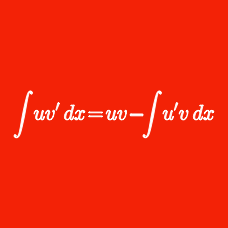Calculus

# Integration with Partial Fractions

If $f(x)=\int \frac{4x}{x^2-2x-3} dx$ and $f(0)=15 \ln 3,$ what is the value of $f(2)?$

If $f(x)$ is a function such that $f'(x)=\frac{1}{25x^2-1}$ and $f(0)=7,$ what is the value of $f(1)?$

What is the indefinite integral $\int \frac{x+2}{(x-4)(x-5)} dx?$ (Use $C$ for the constant of integration.)

If $f(x)=\int \frac{12x^2}{(x-1)(x^2+x+1)} dx$ and $f(0)=0,$ what is the value of $\displaystyle{e^{\frac{f(9)}{4}}}?$

If $a$ is a nonzero real number, what is $\int \frac{35}{x^2 - a^2} dx?$

Details and assumptions

Use $C$ as the constant of integration.

×